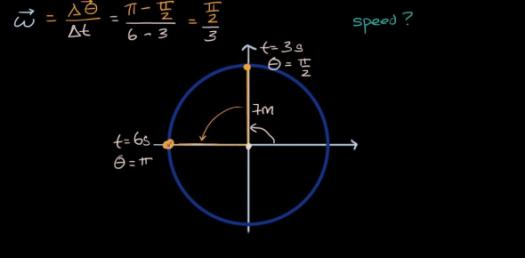# Physics Quiz: Laws Of Motion And Gravitation Questions!

10 Questions | Total Attempts: 2510Settings• 1.
The acceleration in a body is due to ___________
• A.

Balanced force

• B.

Un-balanced force

• C.

Electro static force

• 2.
The physical quantity which is equal to rate of change of momentum is ___________
• A.

Displacement

• B.

Acceleration

• C.

Force

• D.

Impulse

• 3.
The momentum of a massive object at rest is _______
• A.

Very large

• B.

Very small

• C.

Zero

• D.

Infinity

• 4.
The weight of 50 kg person at the surface of earth is ________
• A.

50 N

• B.

35 N

• C.

380 N

• D.

490 N

• 5.
The freezing of biotechnology products like vaccines require ________ freezing systems.
• A.

Helium

• B.

Nitrogen

• C.

Ammonia

• D.

Chlorine

• 6.
From the following statements write down that which is not applicable to mass of an object.
• A.

It is a fundamental quantity.

• B.

It is measured using physical balance.

• C.

It is measured using spring balance.

• 7.
Fill in the blanks. Force = mass x acceleration, then momentum = ____x______
• A.

Weight x velocity

• B.

Mass x speed

• C.

Mass x velocity

• D.

Weight x speed

• 8.
Fill in the blanks. Liquid hydrogen is for rocket, then –––– for MRI.
• A.

Liquid ammonia

• B.

Liquid Helium

• C.

Liquid Hydrogen

• D.

Liquid petroleum

• 9.
The name of some organisations which are associated with Chandrayan-I mission are given below. but some of them are not. List out the wrong ones. ISRO, BARC, NASA, ESA
• A.

ISRO

• B.

BARC

• C.

NASA

• D.

ESA

• 10.
As a matter of convention, an anticlockwise moment is taken as ________ and a clockwise moment is taken as  ________.
• A.

Negative, positive

• B.

Positive, negative

• C.

Positive, positive

• D.

Negative, negative

Related TopicsBack to top